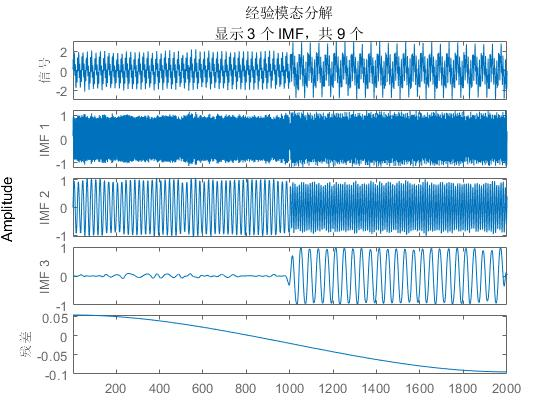• EEMD是Ensemble Empirical Mode Decomposition的缩写，中文是集合经验模态分解，是针对EMD方法的不足，提出了一种噪声辅助数据分析方法。EEMD分解原理是当附加的白噪声均匀分布在整个时频空间时，该时频空间就由...matlab
• 经验模态分解程序，没有采用其他函数，完全按照经验模态分解的流程进行经验模态分解
• 全面的EEMD程序，用作信号分解，故障诊断邻域得到广泛应用
• 内部包含EMD/EEMD/CEEMDAN/VMD的matlab程序代码，可以用于进行信号的经验模态分解
• matlab平台下，采用经验模态分解，对具有突变特征的波形数据进行分析，以准确获取突变点EMD matlab
• EMD经验模态分解MATLAB程序包，将压缩包中的文件夹添加在MATLAB的子路径之下，然后在MATLAB面板上方点击Set path→添加子路径，在编程时调用emd()即可使用
• 一：下载EMD的MATLAB程序和MATLAB的时频工具包EMD的MATLAB程序下载地址：http://perso.ens-lyon.fr/patrick.flandrin/emd.htmlMATLAB程序的作者法国人Rilling的程序写的相关的文章：《on empirical mode decompos ...

一：下载EMD的MATLAB程序和MATLAB的时频工具包

EMD的MATLAB程序下载地址：http://perso.ens-lyon.fr/patrick.flandrin/emd.html

MATLAB程序的作者法国人Rilling的程序写的相关的文章：《on empirical mode decompos and its algorithms》

EMD的程序要用到matlab的时频工具箱，工具箱下载地址：http://tftb.nongnu.org/

matlab的工具箱的安装步骤：

1.解压下载的文件包，将tft/mfile复制到matlab的toolbox文件夹下

->选择mfile文件夹复制到matlab的toolbox的路径->save->close

3.测试是否建立搜索路径成功，在matlab命令窗口打入：which fmsin

返回的结果是你放mfile的正确路径说明建立搜索路径成功。此时就完成了安装工具包的工作。

此时EMD的准备工作就完了。

二：

新建一个文件夹，在此文件下编写你自己的程序，并把需要的EMD程序需要的m文件都拷贝到这个编程的文件夹下。

matlab里的current directroy当前工作目录选到你的编程目录。

1.测试：《on empirical mode decompos and its algorithms》图1的程序

需要的文件:

emd_fmsin.m

emd.m(io.m)

emd_visu.m

展开全文• LMD经验模态分解matlab程序LMD经验模态分解matlab程序——原味的曾经也用滑动平均写过LMD，其实滑动平均的EMD才是原汁原味的居于均值分解。分享给有需要的人，程序写的不好，只是希望提供一种思路。如果谁写了更完美...

LMD经验模态分解matlab程序

LMD经验模态分解matlab程序——原味的

曾经也用滑动平均写过LMD，其实滑动平均的EMD才是原汁原味的居于均值分解。

分享给有需要的人，程序写的不好，只是希望提供一种思路。如果谁写了更完美LMD程序，别忘了发我一份，快毕业了，一直没有把LMD写完美，对于我来说始终是个遗憾。来分完美的LMD让我也品尝下，我也无憾了~

代码下载地址：/source/3102096

此处没有提供测试代码，如需要可以点这里：点我

源代码如下：

%原始lmd算法，效果很不好，不知道程序哪里写错function[PF,A,SI]=lmd(m)c=m;k=0wucha1=0.001;n_l=nengliang(m);while 1????k=k+1;????a=1;????h=c;????[pf,a,si]=zhaochun(a,h,wucha1);????c=c-pf;????PF(k,:)=pf;????A(k,:)=a;????SI(k,:)=si;????c_pos=pos(c);????n_c=nengliang(c);????n_pf=nengliang(pf);????if length(c_pos)<3 || n_c

function pos=pos(y)%功能：找序列极值点位置坐标

%y:输入序列%pos:极值点的序列位置坐标m = length(y);d = diff(y);

n = length(d);d1 = d(1:n-1);d2 = d(2:n);indmin = find(d1.*d2<0 & d1<0)+1;indmax = find(d1.*d2<0 & d1>0)+1;

if any(d==0)???imax = [];??imin = [];???bad = (d==0);??dd = diff([0 bad 0]);??debs = find(dd == 1);??fins = find(dd == -1);??if debs(1) == 1????if length(debs) > 1??????debs = debs(2:end);??????fins = fins(2:end);????else??????debs = [];??????fins = [];????end??end??if length(debs) > 0????if fins(end) == m??????if length(debs) > 1????????debs = debs(1:(end-1));????????fins = fins(1:(end-1));

??????else????????debs = [];????????fins = [];??????end?????????end??end??lc = length(debs);??if lc > 0????for k = 1:lc??????if d(debs(k)-1) > 0????????if d(fins(k)) < 0??????????imax = [imax round((fins(k)+debs(k))/2)];????????end??????else????????if d(fins(k)) > 0??????????imin = [imin round((fins(k)+debs(k))/2)];????????end??????end????end??end???if length(imax) > 0????indmax = sort([indmax imax]);??end

??if length(imin) > 0????indmin = sort([indmin imin]);??end?end?

minmax=cat(2,indmin,indmax);pos=sort(minmax);end%----------zhaochun.mfunction [pf,a,si]=zhaochun(a,h,wucha1)chun_num=0;while 1chun_num=chun_num+1t=1:length(h);h_pos=position(h);%极值点位置序列tpoint=t(h_pos);%极值点时间值hpoint=h(h_pos);%极值点幅度值hpoint

展开全文• MATLAB背景下的改进经验模态分解程序，适合入门学习，欢迎下载。
• 对非平稳信号进行经验模态分解，提取IMF，并进行HILBERT变换。很好的程序
• 经验模态分解是2000年以来以傅立叶变换为基础的线性和稳态频谱分析的一个重大突破，它是依据信号自身的时间尺度特征对信号进行分解，无需预先设定任何基函数，这一点与建立在先验性的谐波基函数和小波基函数上的傅立...

mi=(al+ar)/2;

ai=abs(ar-al)/2;

end

function c=junzhi(a)

l=length(a);

al=wkeep(a,l-1,'l');

ar=wkeep(a,l-1,'r');

c=(al+ar)/2;

end

d=[a';c';b']';

%头：尾：中间

end

%输入参数a:点序列(行向量)(包含端点和极值点以 在时间上的位置-横坐标)(n个)(点序列-横坐标)

%输入参数b:段序列(行向量)(点序列a 每两点之间的纵坐标的值-纵坐标) (n-1)-(段序列-纵坐标)

%输入参数c:点序列(行向量) (包含端点和极值点 在对应时间上的幅值-纵坐标)(n个)-(点序列-纵坐标)

%输入参数d:原始数据的采样精度

%输出参数f_value(行向量): 点序列a 插入 段序列的值 之后，以c的精度的 值(对应于 横坐标，纵坐标的值)

%精度是0.001

l=length(b);

al=wkeep(a,l,'l');

ar=wkeep(a,l,'r');

d={[]};%d={0}这样是为了初始化 元胞数组

for i=1:l %采样精度0.001

d{i}=ones(1,(ar(i)-al(i)-1))*b(i);%ones函数参数要为整数，unint16就是数据强制类型转换，

end %这里没有使用到单独为uint16((ar(i)-al(i))*1000)-1)这个自变量赋值，所以只是个中间变量，对数据不会产生污染

y=c(1);

for i=1:l

end

f_value=y;

end

end

%------

function p=nengliang(y)

% my=mean(y);

% p=(y-my).*(y-my);

% p=sum(p);

p=sum(abs(y).^2);

end

%--------

展开全文• 经验模态分解及希尔伯特频谱分析主程序，有注释，为了提高程序适用性多设了输入输出变量，根据实际情况可以删减
• 基于经验模态分解（EMD）去噪的matlab代码,(Denoising signals using empirical mode decomposition and hurst analysis)
• 利用经验模态分解提取IMF向量与残余向量的matlab程序，可以直接运行emdplus.m，亲测有效，不能运行不要钱~，主函数与样本熵函数samp经过了分析，里面有详细注解，EMD.m函数用的歪国人写的的~目前也是较为常用的版本...
• MATLAB自带函数实现经验模态分解 MATLAB从2018a开始给出了内置函数来实现经验模式分解（EMD）与希尔伯特-黄变换（HHT），函数名分别是emd与hht，用户可以直接调用两个函数来实现经验模式分解以及与希尔伯特-黄变换。...

MATLAB自带函数实现经验模态分解

MATLAB从2018a开始给出了内置函数来实现经验模式分解（EMD）与希尔伯特-黄变换（HHT），函数名分别是emd与hht，用户可以直接调用两个函数来实现经验模式分解以及与希尔伯特-黄变换。

Syntax(语法)

1. [imf,residual] = emd(X)

returns intrinsic mode functions imf and residual signal residual corresponding to the empirical mode decomposition of X. Use emd to decompose and simplify complicated signals into a finite number of intrinsic mode functions required to perform Hilbert spectral analysis.

意思就是返回值是一个经过emd处理后的本征函数imf和残差信号。验证一下，把imf和残差信号相加就是你输入的那个信号。

2. [imf,residual,info] = emd(X)

returns additional information info on IMFs and residual signal for diagnostic purposes.

3. [___] = emd(___,Name,Value)

performs the empirical mode decomposition with additional options specified by one or more Name,Value pair arguments.

类似键值对的输入方式，先输入键名，再输入值。

4. emd(___)

plots the original signal, IMFs, and residual signal as subplots in the same figure.

Matlab里面的例子

1.对信号进行经验模态分解和希尔伯特谱可视化
t = (0:length(X)-1)/fs;
plot(t,X)
xlabel(‘Time(s)’)

sinusoidalSignalExampleData.mat这个数据在matlab文件里，使用命令help emd，然后进入说明文档那里，点开OpenLive Script就有了。

混合信号包含不同振幅和频率值的正弦波。

要创建希尔伯特频谱图，需要信号的固有模态函数(IMFs)。进行经验模态分解，计算信号的imf和残差。由于信号不是平滑的，指定’pchip’作为插值方法。

[imf,residual,info] = emd(X,‘Interpolation’,‘pchip’);

这条命令出来的结果是多了一个info的1×1struct，里面有6个字段，分别是NumIMF，NumExtrema,NumZerocrossing,NumSifting,MeanEnvelopeEnergy和RelativeTolerance。

使用经验模态分解得到的imf分量创建希尔伯特谱图。 命令：hht(imf,fs)

频率与时间的关系图是一个稀疏的图，其中有一个竖直的彩色条，表示IMF中每个点的瞬时能量。图中是原始混合信号分解后各分量的瞬时频谱。三个imf出现在图中，其频率在1秒内发生明显变化。

2.正弦波本征模态函数的过零和极值

生成两个正弦波s和z，这样s是三个正弦波的和，z是一个调幅的单一正弦波。通过计算两个信号差的无穷范数来验证两个信号是相等的。

t = 0:1e-3:10;
omega1 = 2*pi*100;
omega2 = 2*pi*20;
s = 0.25*cos((omega1-omega2)*t) + 2.5*cos(omega1*t) + 0.25*cos((omega1+omega2)*t);
z = (2+cos(omega2/2*t).^2).*cos(omega1*t);
norm(s-z,Inf)

ans = 3.2729e-13

绘制正弦曲线并选择从2秒开始的1秒间隔。

plot(t,[s' z'])
xlim([2 3])
xlabel('Time (s)')
ylabel('Signal')

获取信号的声谱图。谱图显示了三个不同的正弦分量。傅里叶分析把这些信号看作是正弦波的叠加。

使用emd来计算信号的固有模态函数(IMFs)和附加的诊断信息。默认情况下，该函数输出一个表，其中指示每个IMF的筛选迭代次数、相对容忍度和筛选停止条件。经验模态分解把信号看成z。

零交叉和局部极值的数目最多相差1。这满足了信号为IMF的必要条件。

绘制IMF曲线，并选择从2秒开始的0.5秒间隔。IMF是一个AM信号，因为emd认为信号是调幅的

plot(t,imf)
xlim([2 2.5])
xlabel('Time (s)')
ylabel('IMF')

3.计算振动信号的固有模态函数
略（目前好像用不着）

4.可视化信号的残差和本征模态函数
加载并可视化一个由频率变化明显的正弦波组成的非平稳连续信号。电钻的振动和烟花的声音都是非平稳连续信号的例子。

t = (0:length(X)-1)/fs;
plot(t,X)
xlabel('Time(s)')

混合信号包含不同振幅和频率值的正弦波。

进行经验模态分解，绘制信号的本征模态函数和残差。由于信号不是平滑的，指定’pchip’作为插值方法。

命令：emd(X,‘Interpolation’,‘pchip’,‘Display’,1)

Current IMF  |  #Sift Iter  |  Relative Tol  |  Stop Criterion Hit
1      |        2     |     0.026352   |  SiftMaxRelativeTolerance
2      |        2     |    0.0039573   |  SiftMaxRelativeTolerance
3      |        1     |     0.024838   |  SiftMaxRelativeTolerance
4      |        2     |      0.05929   |  SiftMaxRelativeTolerance
5      |        2     |      0.11317   |  SiftMaxRelativeTolerance
6      |        2     |      0.12599   |  SiftMaxRelativeTolerance
7      |        2     |      0.13802   |  SiftMaxRelativeTolerance
8      |        3     |      0.15937   |  SiftMaxRelativeTolerance
9      |        2     |      0.15923   |  SiftMaxRelativeTolerance

The decomposition stopped because the number of extrema of the residual signal is less than ‘MaxNumExtrema’.上面这个命令给出了一个迭代次数的表格，还有一个Figure：经验模态分解的各imf分量，但是图中只显示3个imf分量和1个残差，如果要查看所有imf分量，就要用鼠标在途中右键，选择imf选择器。

展开全文1024程序员节 matlab 信号处理
• 曾经也用滑动平均写过LMD，其实滑动平均的EMD才是原汁原味的居于均值分解。分享给有需要的人，程序写的不好，只是希望提供一种思路。如果谁写了更完美LMD程序，别忘了发我一份，快毕业了，一直没有把LMD写完美，对于...
• 该代码为信号分解中的经验模态分解技术的Matlab实现，该技术能够用于地震勘探中地震信号的去噪处理以及时频分析的研究，具有非常广泛的应用前景，目前，该技术得到了进一步发展，该程序可作为该类技术研究的基础
• 包含IMF本征模函数，包含信号频谱分析等详细代码，有详细注释程序
• 一、使用MATLAB自带函数 如果你的MATLAB版本是2018a及更新版本，那么是可以直接调用emd函数的。 以下代码在MATLAB2019a中编写，未在其他版本中测试。 load('sinusoidalSignalExampleData.mat','X','fs') %载入...
• % 关闭图形 if display_sifting close end end % % 测试是否存在足够的极值点（3个）进行分解，极值点个数小于3个则返回1，这是整体停止条件 function stop = stop_EMD(r,MODE_COMPLEX,ndirs) if MODE_COMPLEX % 复...
• 提出的多元经验模式分解的新算法，matlab程序，适合多元数据的联合分析与数据级的多通道数据融合，是一种非常具有前景的一种算法。
• MATLAB背景下的复杂经验模态分解程序，适合入门学习，欢迎下载。
• 一：下载EMD的MATLAB程序和MATLAB的时频工具包MATLAB程序的作者法国人Rilling的程序写的相关的文章：《on empirical mode decompos andits algorithms》EMD的程序要用到matlab的时频工具箱，工具箱下载地址：...
• 经验模态分EMD的改进CEEMDAN方法源代码，可以直接下载后调用
• 经验模态分解程序，用于信号分解经验模态分解(Empirical Mode Decomposition，简称EMD))方法被认为是2000年来以傅立叶变换为基础的线性和稳态频谱分析的一个重大突破，该方法是依据数据自身的时间尺度特征来进行信号...
• 能够可以运行的关于matlab中的局部均值分解（LMD）的完整程序matlab
• 这是经验模式分解(EMD)的另一种Python实现。该包包含许多EMD变体，并打算及时交付更多。 from PyEMD import EMD import numpy as np s = np.random.random(100) emd = EMD() IMFs = emd(s) ......

经验模态分解matlab程序matlab 订阅